# Analytical reasoning test-2

16)

In each of the the following question, count the number of triangles and squares in the given figure.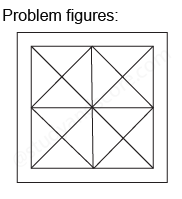17)

In each of the the following question, count the number of triangles and squares in the given figure.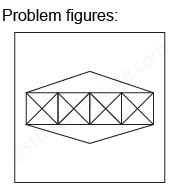18)

In each of the the following question, count the number of triangles and squares in the given figure.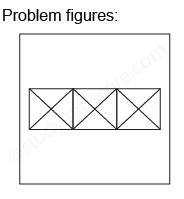19)

In the adjoining figure if the centres of all the circles are joined by horizontal and vertical lines, then find the number of squares that can formed.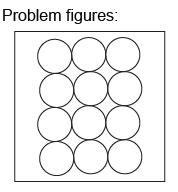20)

In each of the the following question, count the number of triangles and squares in the given figure.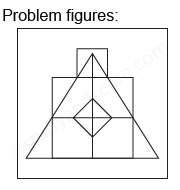--Share with your friends --